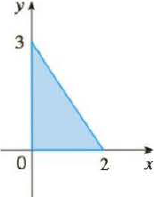Chapter 8.3, Problem 34E

Chapter
Section
Textbook Problem

Calculate the moments Mx and My and the center of mass of a lamina with the given density and shape.34. ρ = 4To determine

To find: The moments Mx, My and the center of mass of a lamina with the given density and shape.

Explanation

Given:

The density is ρ=4

Calculation:

Calculate the area of given shape:

A=12bh (1)

Substitute 2 for b and 3 for h in Equation (1).

A=12×2×3=3

Calculate the mass of given shape:

m=ρA (2)

Substitute 4 for ρ and 3 for A in Equation (2).

m=4×3=12

Consider y=32x+3

Calculate the moment Mx of a lamina with the given density and shape:

Mx=ρab12y2dx (3)

Substitute 0 for a, 2 for b, 4 for ρ, and (32x+3) for y in Equation (3).

Mx=40212(32x+3)2dx=4×1202(94x29x+9)dx=2×902(x24x+1)dx=1802(x24x+1)dx (4)

Integrate Equation (4).

Mx=18[x2+14(2+1)x1+11+1+x]02=18[x312x22+x]02=18{[(2)312(2)22+(2)][(0)312(0)22+(0)]}

=18(462+2)0=12

Hence, the moment Mx of a lamina with the given density and shape is 12.

Calculate the moment My of a lamina with the given density and shape:

My=ρabxydx (5)

Substitute 0 for a, 2 for b, 4 for ρ, and (32x+3) for y in Equation (5)

Still sussing out bartleby?

Check out a sample textbook solution.

See a sample solution

The Solution to Your Study Problems

Bartleby provides explanations to thousands of textbook problems written by our experts, many with advanced degrees!

Get Started

Differentiate f and find the domain of f. f(x) ln ln ln x

Single Variable Calculus: Early Transcendentals, Volume I

Simplify the expressions in Exercises 97106. 21/a/22/a

Finite Mathematics and Applied Calculus (MindTap Course List)

In Exercises 2340, find the indicated limit. 38. limx22x3+4x2+1

Applied Calculus for the Managerial, Life, and Social Sciences: A Brief Approach

In Problems 7-34, perform the indicated operations and simplify. 10.

Mathematical Applications for the Management, Life, and Social Sciences

True or False: converges if and only if .

Study Guide for Stewart's Multivariable Calculus, 8th

Describe how replication protects against fraud being committed in research.

Research Methods for the Behavioral Sciences (MindTap Course List)